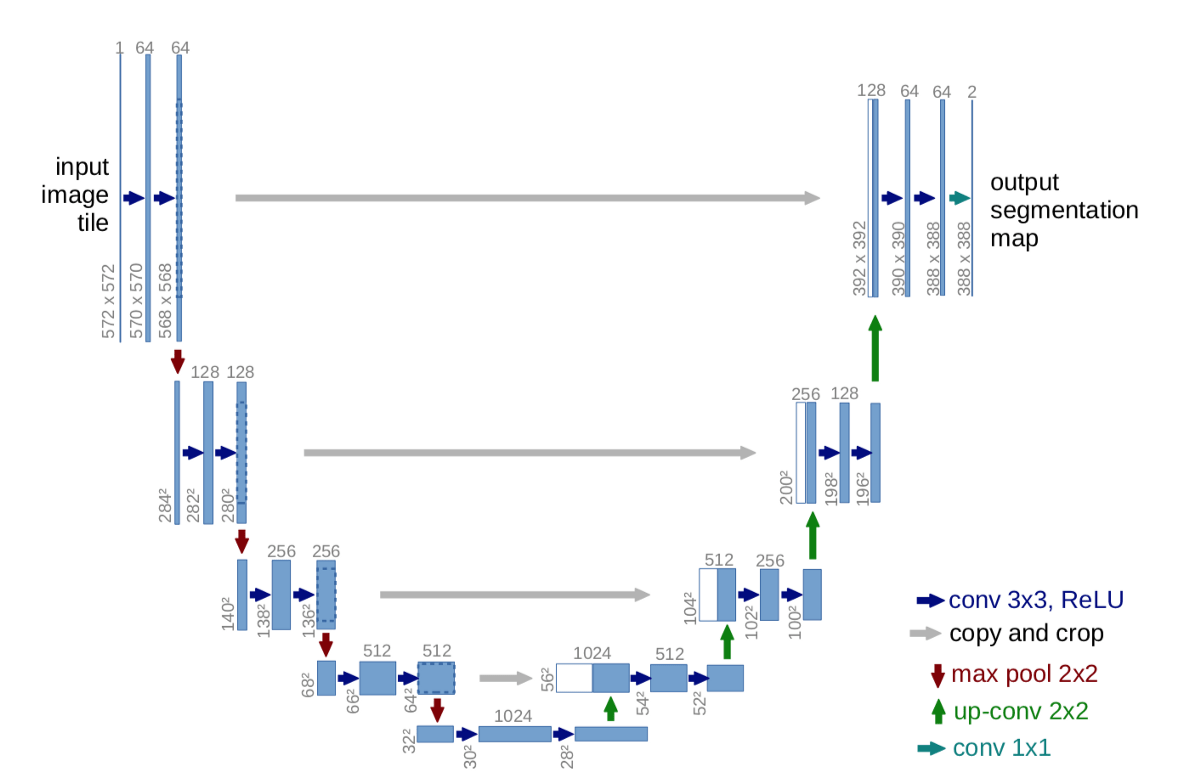# Given transposed=1, weight of size [1024, 512, 2, 2], expected input[5, 1536, 20, 20] to have 1024 channels, but got 1536 channels instead

I am trying to implement UNET from scratch using this image.This is my code:

``````class DoubleConv2D(nn.Module):
def __init__(self, in_channels, out_channels):
super(DoubleConv2D, self).__init__()
self.in_channels = in_channels # 3
self.out_channels = out_channels # 1
self.conv2d = nn.Sequential(
nn.Conv2d(self.in_channels, self.out_channels, 3, 1, 1, bias=False), # padding = 1 = same convolution = in h,w will be same after conv operation
nn.BatchNorm2d(self.out_channels),
nn.ReLU(inplace=True),
nn.Conv2d(self.out_channels, self.out_channels, 3, 1, 1, bias=False),
nn.BatchNorm2d(self.out_channels),
nn.ReLU(inplace=True)
)
def forward(self, x):
return self.conv2d(x)

class UNET(nn.Module):
def __init__(self, in_channels=3, out_channels=1, filters=[64, 128, 256, 512]):
super(UNET, self).__init__()
self.in_channels = in_channels
self.out_channels = out_channels
self.filters = filters

self.up_sample_layers = nn.ModuleList()
self.down_sample_layers = nn.ModuleList()
self.maxpool = nn.MaxPool2d(2, 2)

# downsampling
for filter_channel in self.filters:
self.down_sample_layers.append(DoubleConv2D(self.in_channels, filter_channel)) # 3 to 64
self.in_channels = filter_channel # 3 = 64

self.bottleneck = DoubleConv2D(self.filters[-1], self.filters[-1]*2)

# upsampling
for filter_channel in reversed(self.filters):
self.up_sample_layers.append(nn.ConvTranspose2d(filter_channel*2, filter_channel, kernel_size=2, stride=2))
self.up_sample_layers.append(DoubleConv2D(filter_channel*2, filter_channel))

self.final_conv = nn.Conv2d(self.filters, self.out_channels, kernel_size=1)

def forward(self, x):
skip_connections = []

# downsampling
for down_sampling_layer in self.down_sample_layers:
x = down_sampling_layer(x)
# save skip connection for later use before applying max pool layer
skip_connections.append(x)
x = self.maxpool(x)

x = self.bottleneck(x)

# reversing skip connections list because we need to go in opposite direction
skip_connections = skip_connections[::-1]
print([t.shape for t in skip_connections])

# upsampling
for index, up_sampling_layer in enumerate(self.up_sample_layers):
if index%2:
x = up_sampling_layer(x)
else:
skip_connection = skip_connections[index]
if x.shape != skip_connection.shape:
x = F.resize(x, size=skip_connection.shape[2:])
print(x.shape)
print(skip_connection.shape)
concat = torch.cat((skip_connection, x), dim=1) # dim=1 = channels, (batch, c, h, w)
#ERROR
x = up_sampling_layer(concat)

return self.final_conv(x)
``````

Output:

``````[torch.Size([5, 512, 20, 20]), torch.Size([5, 256, 40, 40]), torch.Size([5, 128, 80, 80]), torch.Size([5, 64, 160, 160])]
torch.Size([5, 1024, 20, 20])
torch.Size([5, 512, 20, 20])

---------------------------------------------------------------------------

RuntimeError                              Traceback (most recent call last)

<ipython-input-23-ceca97b4e23f> in <module>()
2 input_sample = torch.randn((5, 3, 160, 160)) # batch, channels, height, width
3 model = UNET(in_channels=3, out_channels=1)
----> 4 predictions = model(input_sample)
5 print(input_sample.shape)
6 print(predictions.shape)

3 frames

/usr/local/lib/python3.7/dist-packages/torch/nn/modules/module.py in _call_impl(self, *input, **kwargs)
1049         if not (self._backward_hooks or self._forward_hooks or self._forward_pre_hooks or _global_backward_hooks
1050                 or _global_forward_hooks or _global_forward_pre_hooks):
-> 1051             return forward_call(*input, **kwargs)
1052         # Do not call functions when jit is used
1053         full_backward_hooks, non_full_backward_hooks = [], []

<ipython-input-22-6a212bc6c9a5> in forward(self, x)
52         concat = torch.cat((skip_connection, x), dim=1) # dim=1 = channels, (batch, c, h, w)
53         #ERROR
---> 54         x = up_sampling_layer(concat)
55
56     return self.final_conv(x)

/usr/local/lib/python3.7/dist-packages/torch/nn/modules/module.py in _call_impl(self, *input, **kwargs)
1049         if not (self._backward_hooks or self._forward_hooks or self._forward_pre_hooks or _global_backward_hooks
1050                 or _global_forward_hooks or _global_forward_pre_hooks):
-> 1051             return forward_call(*input, **kwargs)
1052         # Do not call functions when jit is used
1053         full_backward_hooks, non_full_backward_hooks = [], []

/usr/local/lib/python3.7/dist-packages/torch/nn/modules/conv.py in forward(self, input, output_size)
916         return F.conv_transpose2d(
917             input, self.weight, self.bias, self.stride, self.padding,